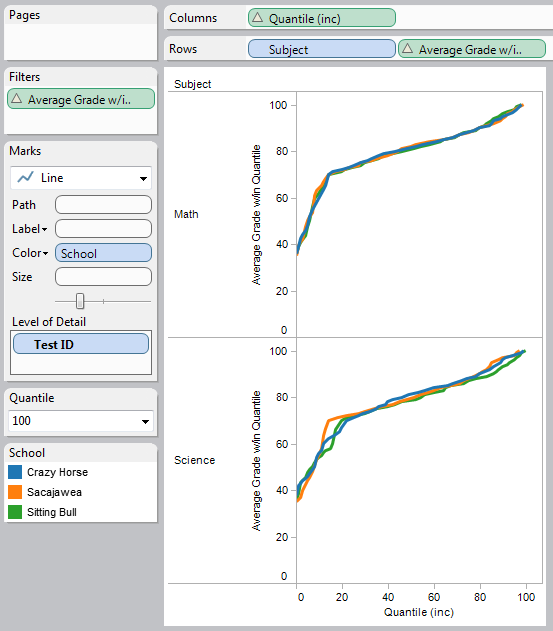Analyses

This section covers how to generate different types of analyses in Tableau. For example, how to do your own projections and forecasts (a lot of that material comes from Tableau v7 and earlier), some cohort analysis, etc.

Be sure to check out the following sources of material on the Tableau Community:

Projections

Forecasting, Run Rate and Projected Visits:

projections – iterative over months, messy
http://community.tableausoftware.com/message/178156
file is: Projection Sample jtd edit.twbx

projections – easy linear trend
file is: Forecast jtd edit.twbx

BYO Optimal Trend Line using table calcs

http://community.tableausoftware.com/message/180906#180906
workbook is Optimal Trend Line.twbx

Duplicate data to do funky action filter where the filtered data still shows difference from current to previous
http://community.tableausoftware.com/message/174252#174252

Comparing dimensions

Also check out Sets, lots of possibilities there.

Comparing different dimensions (surveys, etc.)
http://kb.tableausoftware.com/articles/knowledgebase/calculating-percent-difference-across-dimensions-60

comparing items via table calc across columns:
http://kb.tableausoftware.com/articles/knowledgebase/comparing-items

Find complementary products- if customer purchased X, see what else the purchased

http://community.tableausoftware.com/message/171706#171706\

Compare one against the rest

http://kb.tableausoftware.com/articles/knowledgebase/comparing-items

http://interworks.co.uk/andy-cotgreave/us-v-the-rest/

One vs. all other

Correlation Matrix by Bora Beran

compare given period  (quarter, month, year, etc.) to the same period some time ago (year or previous)

workbook is date_level_over_year_ago_or_prev.twbx

Quantiles

Big post on percentiles with Tableau:
http://www.equinox.co.nz/blog/Lists/Posts/Post.aspx?ID=160

[A] is the measure
[p] is the quantile, e.g. .25 for 25th, etc.
Set compute using to [A], may need to use and advanced compute to order along the desired field.

Return only one value:

// Only calculate for first row of the partition, otherwise return NULL
IF (FIRST()==0) THEN
// p = 0 => minimum, and we are on the first row, so we just want the current value
IF ([p] == 0) THEN
ATTR([A])
// p = 1 => maximum, so lookup the last row
ELSEIF ([p] == 1) THEN
LOOKUP(ATTR([A]), LAST())
// otherwise select the appropriate value
ELSE
// The WINDOW_MIN() finds the lowest value at or beyond the required quantile.
WINDOW_MIN(
// required quantile is at the mid-point between two values, so take the average
IF [p] * TOTAL(SUM([Number of Records])) = RUNNING_SUM(SUM([Number of Records])) THEN
(ATTR([A]) + LOOKUP(ATTR([A]), 1)) / 2
// row is beyond required quantile
ELSEIF [p] * TOTAL(SUM([Number of Records])) < RUNNING_SUM(SUM([Number of Records])) THEN
ATTR([A])
ELSE
NULL
END,
0,
IIF(FIRST()==0, LAST(), 0)
)
END

Return same value for all rows, not including 0th or 100th percentile:

PREVIOUS_VALUE(
IF (FIRST()==0) THEN
IF ([p] == 0) THEN
ATTR([A])
ELSEIF ([p] == 1) THEN
LOOKUP(ATTR([A]), LAST())
ELSE
WINDOW_MIN(
IF [p] * TOTAL(SUM([Number of Records])) = RUNNING_SUM(SUM([Number of Records])) THEN
(ATTR([A]) + LOOKUP(ATTR([A]), 1)) / 2
ELSEIF [p] * TOTAL(SUM([Number of Records])) < RUNNING_SUM(SUM([Number of Records])) THEN
ATTR([A])
ELSE
NULL
END,
0,
IIF(FIRST()==0, LAST(), 0)
)
END
END
)

Return same value for all rows, including 0th and 100th percentile:

PREVIOUS_VALUE(
IF (FIRST()==0) THEN
IF ([p] == 0) THEN
ATTR([A])
ELSEIF ([p] == 1) THEN
LOOKUP(ATTR([A]), LAST())
ELSE
WINDOW_MIN(
IF [p] * TOTAL(SUM([Number of Records])) = RUNNING_SUM(SUM([Number of Records])) THEN
(ATTR([A]) + LOOKUP(ATTR([A]), 1)) / 2
ELSEIF [p] * TOTAL(SUM([Number of Records])) < RUNNING_SUM(SUM([Number of Records])) THEN
ATTR([A])
ELSE
NULL
END,
0,
IIF(FIRST()==0, LAST(), 0)
)
END
END
)

Find people who also belong to dimension X

Concatenating a field from multiple rows into one field using PREVIOUS_VALUE

Richard Leeke version:
http://community.tableausoftware.com/message/180164#180164

Alex Kerin version:

find difference between dates, counting weekdays, counting work days
Also having a separate table that identifies working days/holidays and then joining to that

Dynamic anchor date with a  relative date filter
http://voyrapido.tumblr.com/post/22763424744/creating-a-dynamic-anchor-date-with-a-relative-date

Stephen Few’s bandlines in Tableau
http://community.tableausoftware.com/message/198511

For surveys, see Chart Types.

[loop category=”wikicontent” tag=”analyses,forecasting,projection,percentiles,rank,cohort-analysis”]
[field title] – Added [field date]
[content]
[/loop]

Related posts:

[loop tag=”analyses,forecasting,projection,percentiles,rank,cohort-analysis” exclude=”this” relation=”and” compare=”not” taxonomy=”category” value=”wikicontent”]